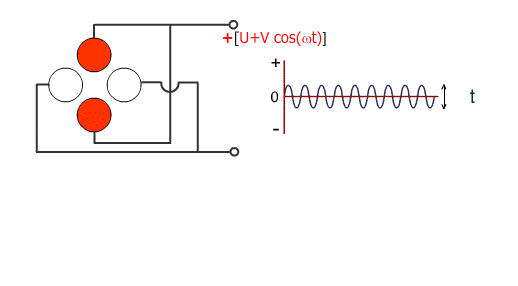# Applied Voltages to Rods

## Voltage is applied to each rod

1. A RF voltage (V cos (wt)) and a DC voltage (U) are simultaneously applied to the facing rods.

2. The RF and DC with the same magnitude but the opposite sign are applied to another pair of rods.

The angular frequency of RF is denoted by w=2pf. The two voltages U and V determine the mass passing through the rods. When U and V voltages are continuously varied with constant U/V, we can obtain the scan data and mass spectrum.